#### Proportional Allocation

When you specify the ALLOC=PROP option in the STRATA statement, PROC SURVEYSELECT allocates the total sample size among the strata in proportion to the stratum sizes, where the stratum size is the number of sampling units in the stratum. The allocation proportion of the total sample size for stratum h equals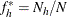where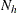is the number of sampling units in stratum h and N is the total number of sampling units for all strata. If you specify the total sample size n in the SAMPSIZE= option in the PROC SURVEYSELECT statement, the procedure computes the target sample size for stratum h as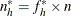The target sample size values,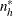, might not be integers, but the stratum sample sizes are required to be integers. PROC SURVEYSELECT uses a rounding algorithm to convert theto integer values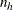and maintain the requested total sample size n. The rounding algorithm includes the restriction that all values ofmust be at least 1, so that at least one unit is selected from each stratum. If you specify a minimum stratum sample size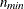in the ALLOCMIN= option in the STRATA statement, then all values ofare required to be at least. For without-replacement selection methods, PROC SURVEYSELECT also requires that each stratum sample size must not exceed the total number of sampling units in the stratum,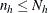. If a target stratum sample size exceeds the number of units in the stratum, PROC SURVEYSELECT allocates the maximum number of units,, to the stratum, and then allocates the remaining total sample size proportionally among the remaining strata.

PROC SURVEYSELECT provides the target allocation proportions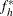in the output data set variable AllocProportion. The variable ActualProportion contains the actual proportions for the allocated sample sizes. For stratum h, the actual proportion is computed as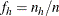whereis the allocated sample size for stratum h and n is the total sample size. The actual proportions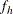can differ from the target allocation proportionsdue to rounding, the requirement that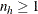(or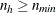), and the requirement thatfor without-replacement selection methods.